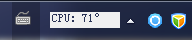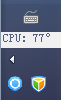## CPU温度小工具之数据呈现

2012年2月2日 2 条评论这个是任务栏在底部的时候我在网上也确实搜索了一把，关于 NET 来实现这样效果的资料好少，年少不学 C++，老来就空叹气。

[DllImport(“user32.dll”, EntryPoint = “FindWindowEx”, SetLastError = true)]
static extern IntPtr FindWindowEx(IntPtr hwndParent, IntPtr hwndChildAfter, string lpszClass, string lpszWindow);

[DllImport(“user32.dll”)]
static extern IntPtr FindWindow(string lpClassName, string lpWindowName);

[DllImport(“user32.dll”)]
static extern int GetWindowRect(IntPtr hwnd, out Rect lpRect);

[DllImport(“user32.dll”, EntryPoint = “MoveWindow”)]
static extern int MoveWindow(IntPtr hwnd, int x, int y, int nWidth, int nHeight, int bRepaint=1);

[DllImport(“user32.dll”)]
static extern int SetParent(IntPtr wnd, IntPtr newParentWnd);

IntPtr trayHwnd = FindWindow(“Shell_TrayWnd”, null); //获取任务栏整体句柄
IntPtr notifyHwnd = FindWindowEx(trayHwnd, IntPtr.Zero, “TrayNotifyWnd”, null);//获取任务栏托盘区域句柄
IntPtr toolbarHwnd = FindWindowEx(trayHwnd, IntPtr.Zero, “ReBarWindow32”, null);//获取任务栏工具条区域句柄
IntPtr imeHwnd = FindWindowEx(toolbarHwnd, IntPtr.Zero, “CiceroUIWndFrame”, null);//获取语言栏区域句柄

Rect box = new Rect();
GetWindowRect(toolbarHwnd, out box);

//获取任务栏尺寸
int boxWidth = box.Right – box.Left;
int boxHeight = box.Bottom – box.Top;
//判断任务栏是水平还是垂直。true 为水平，false 为垂直
bool position = boxWidth > boxHeight ? true : false;

Rect ime = new Rect();
int d = GetWindowRect(imeHwnd, out ime);

//获取语言栏尺寸
int imeWidth = 0;
int imeHeight = 0;
if (d != 0)
{
imeWidth = ime.Right – ime.Left;
imeHeight = ime.Bottom – ime.Top;
}

frmHwnd = FindWindow(null, “SysMonitor”); //查找程序窗体

if (frmHwnd != IntPtr.Zero)
{
int x1, y1; //窗体目标坐标
int x2, y2;//语言栏目标坐标
if (position)
{
x1 = boxWidth – w;
y1 = (boxHeight – h) / 2;

x2 = x1 – imeWidth-10; //这里多减的 10 是为了增加间隙，漂亮点
y2 = 0;
}
else
{
int diff = 2; //垂直方向，扣减宽度更美观
//如果为垂直方向，修改宽度以适应任务栏宽度
w = boxWidth – diff;

x1 = box.Left==0?0:diff;
y1 = boxHeight – h;

x2 = x1;
y2 = boxHeight – imeHeight – h;
}

this.Width = w;
this.Height = h;
lblInfo.Width = w;
lblInfo.Height = h;

int c = SetParent(frmHwnd, toolbarHwnd);//设置任务栏为窗体的父窗体

MoveWindow(frmHwnd, x1, y1, w, h);//移动显示CPU温度的窗体
MoveWindow(imeHwnd, x2, y2, imeWidth, imeHeight);//移动语言栏窗体

CPU 温度工具到这里就算完拉。剩下的一个未完成的是在直接在桌面上绘制内容，相信我，这对我来说很有用的。

## CPU温度小工具之获取温度

2012年2月2日 没有评论

ManagementObjectSearcher search=null;

static string q=”select * from MSAcpi_ThermalZoneTemperature”;

int GetCPUTemperature()
{
search=new ManagementObjectSearcher(“root\\WMI”,q);

ManagementObjectCollection con=search.Get();

foreach (ManagementObject ele in con)
{
Int32 n=Convert.ToInt32(ele.GetPropertyValue(“CurrentTemperature”));
return (n-2732)/10;
}

return 0;
}